﻿ Lorentz force law incompatibility with special relativity

# Solutions to Lorentz force paradox are wrong.

## Solutions to Lorentz force paradox are wrong.

(Fig.1) Special relativity causes fatal paradox.The recent interesting paper ( M. Mansuripur, see this, arxiv, news ) argues that Lorentz force is inconsistent with special relativity.

In the frame of stationary observer (= S ), a neutral wire loop is at rest in the electric field E.
But in the frame of moving observer (= S' ), this wire gets positive or negative charges, and rotates !

This is obviously a paradox, and shows the fatal defects of special realtivity.

(Fig.1') Special relativity failed in Lorentz force.If this result is true, quantum electrodynamics, standard model ( using gauge symmetry ) break down.
So his paper got many objections, as shown on this site.

To begin from the conclusion, as Mansuripur says, special relativity failed in electromagnetism.
As far as I see various objections ( this, this, this ), they depends on "fake" center of rotation, which cannot be solutions to this paradox.

## Charge is attracted to wire ONLY in S' frame ← Paradox !

(Fig.2) Lorentz transformation → new charges (= ρ'e ) are generated ↓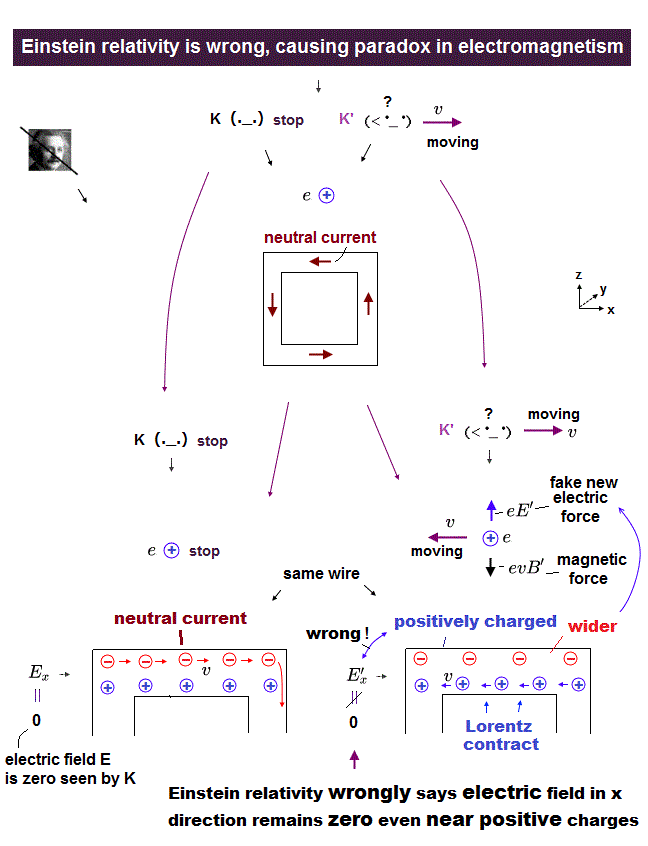According to the special relativity, charge (= ρe ) and electric current (= J ) transform like spacetime (= ct, x, y, z ) under Lorentz transformation.   See websites, this (p.3) this (p.9)

In Fig.2 left, electric wire is neutral (= S ).
But the moment observer starts to move, "positive" charges are generated (= Fig.2 right, S' ) !

As a result, the charged particle (= negative ) outside is attracted toward the wire, ONLY in S' frame.
This is clearly a fatal paradox of relativity.

## Lorentz transformation of charge (= ρ ) and current (= J ).

(Fig.3) Lorentz transformation.Basically, charge (= ρ ) and current (= J ) densities must transform like Fig.3 to satisfy Maxwell equation.
The transformation of Fig.3 is the origin of paradox.

## Neutral wire "rotates" Only by observer's movement ?

(Fig.4) Special relativity fails in Lorentz force.In these papers, they use a loop of the electric wire through which current J is flowing.
This wire contains the same number of positive and negative charges, so it's neutral.

The external electric field E is in the x direction.
As this wire is electrically neutral, so it does NOT rotate by the electric field in the original S frame.

## Neutral wire becomes plus and minus from moving observer !

(Fig.5) Upper wire is plus, lower is minus by observer's movement (= S' ).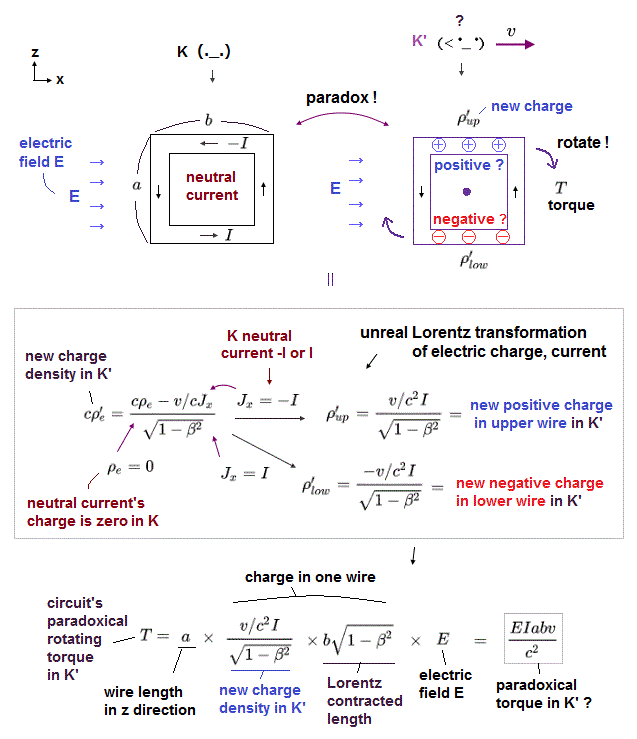In Einstein's unrealistic relativistic world, there are No such things as "absolute space or things", so any concepts (= time, distance, fources, electromagnetic fields, electric charges, masses, energies .. ) must be relatively changed depending on observers' motions.  ← Impossible !

So the electric field and electric charge (= ρ ) are supposed to magically change into the magnetic field and ( neutral ) electric current (= J ) by relativistic Lorentz transformation (= as seen by differently-moving observers,  this p.11 )

According to Lorentz transformation of charge (= ρ ) and current (= J ) densities ( this p.3,  this p.8,  this Box 13.6 ), new strange charges are generated from the viewpoint of moving observer (= S' ) from the originally neutral wire (= S ).

The upper and lower portions of the wire loop carry currents in opposite directions.
So the resultant charges in S' are positive in the upper, negative in the lower wire.

In S frame, the observer is at rest, and the charge density is zero ( ρ = 0 ) due to its neutral wire.
Electric current through the loop is "-J" in the upper, and +J in the lower wire.

In S' frame, the observer is moving at velocity V in the x direction.

Substituting ρ=0 and Jx = -J into Lorentz transformation of Fig.3, the upper wire becomes positive in S' frame !
Substituting ρ=0 and Jx = J into Fig.3, the lower wire becomes negative in S' frame.

## Wire loop rotates only in S' frame. ← Paradox !

(Fig.6) Upper positive wire moves to the right,   lower negative wire moves to the left, in E field.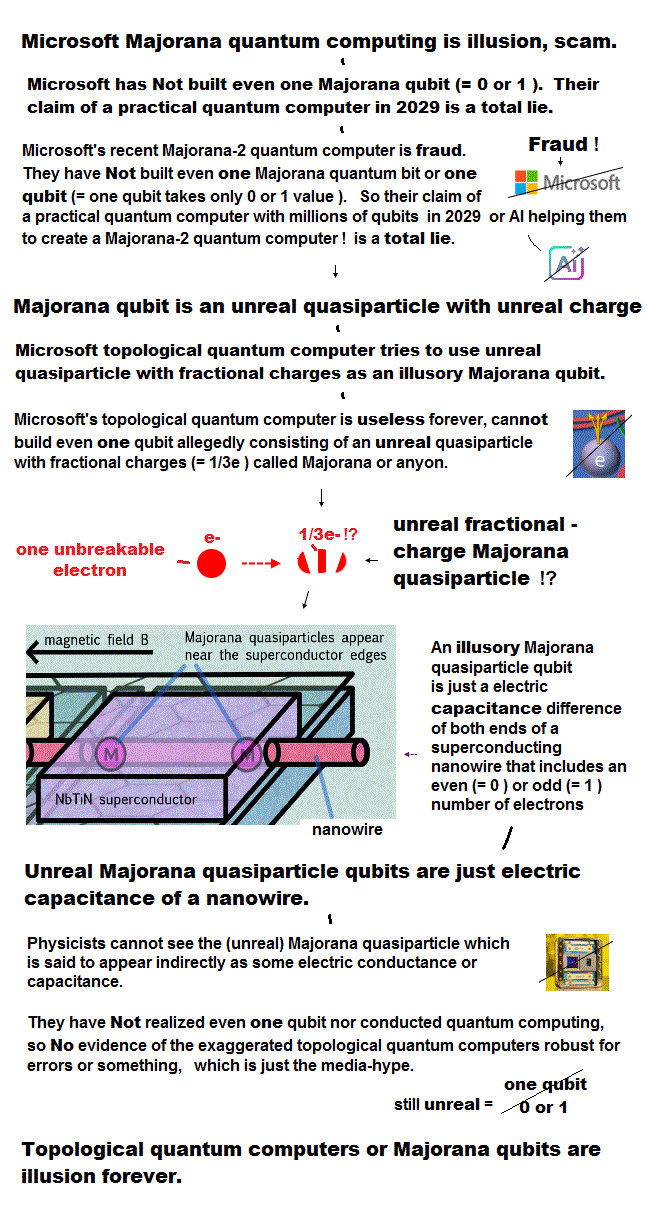As a result, in the electric field E, the upper ( positive ) wire moves to the right, and the lower ( negative ) wire moves to the left in S' frame, so the whole wire rotates clockwise.

In S frame, this wire is neutral, so it does NOT rotate.

Because this observer can rotate this wire just by simple movement (= S' ) without touching it !
Unfortunately, there are NO solutions to this fatal paradox.

## Torque acting on the rotating wire.

(Fig.7) Torque ( N ) = radius (= r ) × force (= F ).Torque (= N ) acting on a thing is equal to the time rate of change of its angular momentum L.
Torque (= moment of force ) is given by radius × force ( N = r × F ).

As shown in Fig.6, the electric field E acts on new generated charges in the upper and lower wire, which causes "torque" ONLY in S' frame.

## "Virtual" rotation center = solution to this paradox ?

(Fig.8) "Virtual" center of rotation = solution ?To solve the paradox of Fig.6, they introduced "virtual" center of rotation. This virtual center is at the point of the moving observer.

And "P" is hidden momentum, which is an artificial concept, too.
Of course, this virtual center has Nothing to do with true center of Fig.7

## "Virtual" center of rotation is NOT real.

(Fig.9) Trick in replacing torque with other things depends on fictitious concepts.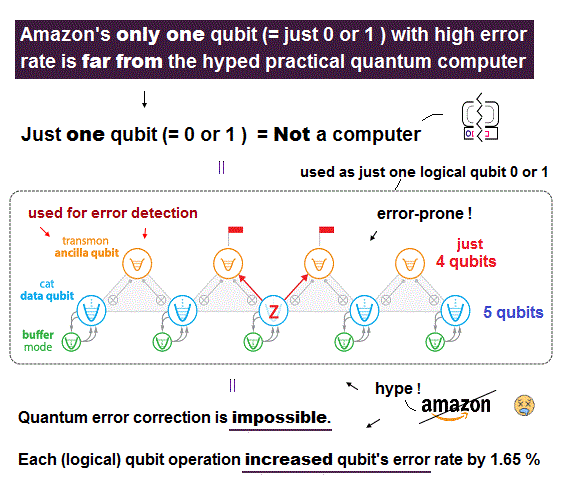Torque ( N = moment of force ) is given by the time derivative of angular momentum (= L ), as shown on this site.
Fig.9 upper (= 1 ) shows the electric field E rotates the whole wire in S' frame (= Fig.6,7 ).

They replace the meaning of this torque by completely different one ( N = time derivative of radius × momentum ), as shown in Fig.9 lower.

But in these solutions ( this, this ), they create "fictitious" center of rotation at the point of the moving observer.
Of course, there is NO real rotation center at this point,

Furthermore, this momentum P is also fictitious hidden momentum.
Depending on these concepts such as illusory rotation center and momentum means Mansuripur's paradox has NOT been solved.

## Virtual radius increases → angular momentum increases ?

(Fig.10) Torque is the time derivative of radius (= r ) × constant P ?They replace the original definition of torque ( N = r × F ) by ( N = the time derivative of r × P ), as shown in Fig.9.
Furthermore, they introduced fictitious center of rotation at the observer moving at velocity V.

The time derivative of fictitious radius ( r = Vt ) is "V", so this torque (?) is equal to V × P (= hidden momentum ), they insist

If this artificial definition is true, the angular momentum can be increased just by observer's uniform motion without external force !
This idea is completely inconsistent with basic physical rule.

## Lorentz force paradox has NOT been solved.

(Fig.11) Virtual center of rotation is NOT real solution.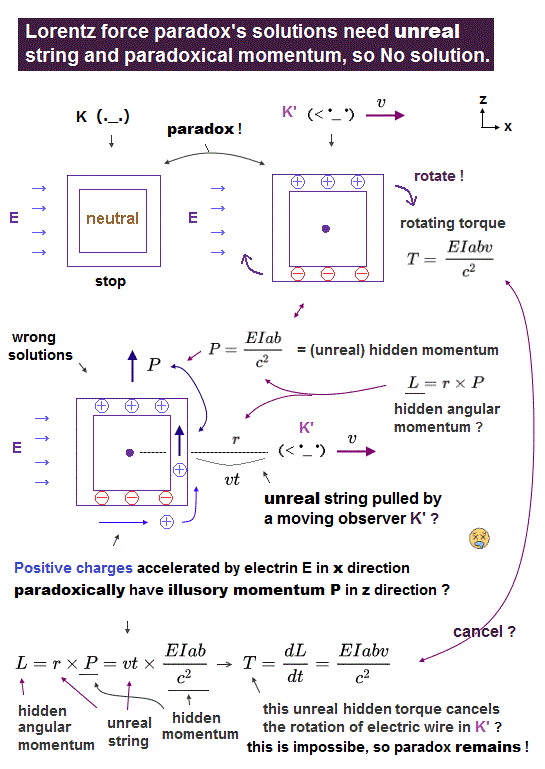As a result, paradox of Lorentz force law has NOT been solved.
Virtual center of rotation has Nothing to do with the original torque.

Because the observer (= virtual center ) is NOT connected to the wire loop by any force or strings.

Various solutions to these true paradox depends on wrong artificial definitions.
Correct solutions must obey basic physical rules.

## Calculating hidden momentum and paradox solution.

(Fig.12) Paradox of Lorentz force ↓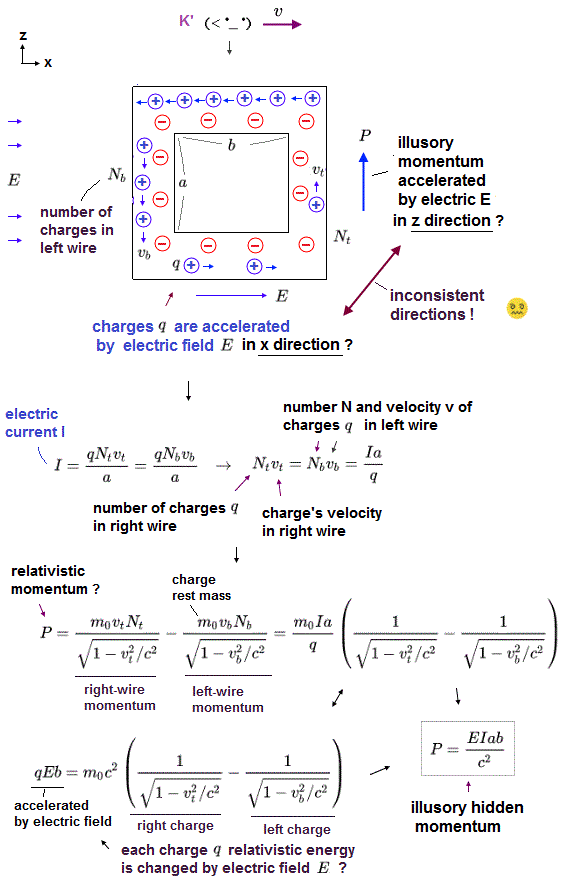In this section, we calculate values of generated charges and torque caused by electric field E.
And we show the original torque is accidentally equal to V × hidden momentum P, as shown in solutions of various papers.

This hidden momentum is also fictitious momentum.
In fact, this hidden momentum depends on wrong physical definitions, which means Lorentz force paradox has NO solutions now.

## Neutral wire is at rest in S frame.

(Fig.13) Neutral wire is at rest from stationary observer (= S ).As seen by stationary observer (= S frame ), the neutral wire loop (= a square with one side "a" in length ) is at rest in the presence of electric field E.

This wire loop is in x-y plane, and the electric field E is in the x direction.
The electric current through the upper ( lower ) portion of this wire flows in -x ( x ) direction.

## Magnetic moment μ = current (= I ) × area (= S ).

(Fig.14) Magnetic moment μ = current I × a2.According to the Maxwell law, the electric current loop causes magnetic dipole moment (= μ ).

This magnetic moment is given by the product of the current I and the loop area S.
In this case, the area S = a2, so μ = Ia2.

## Calculating torque acting on the whole wire.

(Fig.15) New positive and negative charges are generated by observer's movement !In Einstein's unrealistic relativistic world, there are No such things as "absolute space or things", so any concepts (= time, distance, fources, electromagnetic fields, electric charges, masses, energies .. ) must be relatively changed depending on observers' motions.  ← Impossible !

So the electric field and electric charge (= ρ ) are supposed to magically change into the magnetic field and ( neutral ) electric current (= J ) by relativistic Lorentz transformation (= as seen by differently-moving observers,  this p.11 )

According to Lorentz transformation of charge (= ρ ) and current (= J ) densities ( this p.3,  this p.8,  this Box 13.6 ), new strange charges are generated from the viewpoint of moving observer (= S' ) from the originally neutral wire (= S ).

The upper and lower portions of the wire loop carry currents in opposite directions.
So the resultant charges in S' are positive in the upper, negative in the lower wire.

## Paradoxes of Lorentz force law.

(Fig.16) The wire at rest in S frame rotates in S' frame ?   ← Paradox !As a result, though this neutral wire is at rest in S frame, it rotates only in S' frame due to new generated charges.

This is clearly a fatal paradox of relativity.
This paradox has NO solutions, either, when a charge outside is moving, instead of the wire.

ρ' is the charge ( linear ) density in S' frame.

(Fig.17) Torque on the whole wire.Total charge included in each upper and lower wire is given by charge density (= ρ' ) × the legnth of the wire, which is Lorentz contracted.

As a result, total charge in each wire becomes ± VJa/c2.
(Fig.18)The electric force F is given by the total charge × the electric field E.
As the upper and lower wires have the opposite charges, the whole wire rotates only in S' frame !

## Torque (= N ) on the wire.

(Fig.19) Torque on the whole wire is force F × radius.The electric force F in the opposite directions causes torque (= N ) on this whole wire.
This torque N is given by the force F × the distace (= 1/2 a ) from the axis of rotation.

(Fig.20) Total torque (= N )Summing the torque on positive and negative charges ( 1/2a × 2 = a ), the calculated result becomes Fig.19,20 ( using the magnetic moment μ of Fig.14 ).

(Fig.21) Torque (= N ) increases angular momentum (= L ).As shown in Fig.19, torque (= N ) increases the angular momentum (= L ).
In Fig.16,17, the distance (= r = 1/2a ) between force and rotation axis is the constant value.

So the velocity of rotation increases by this torque.

(Fig.22) Torque = time derivative of angular momentum (= L ).Torque (= N ) is given by the time derivative of the angular momentum (= L ).
In this case, the radius r is fixed (= 1/2a ).

So the torque is equal to r × F (= force ), which is a normal form.

## ↓ Wrong tricks used in solutions to paradox.

(Fig.23) ↓ Is this real torque ?As I said, the torque N is the time derivative of the angular momentum.
But they try to change (= differentiate ) the radius ( r = Vt ) instead of the momentum (= P ).

This is clearly wrong trick.
As you see Fig.16, and Fig.17, the radius of rotation is the fixed value ( r= 1/2a in this case ).

So the time derivative of the radius becomes zero in the right answer.

(Fig.24) This is really torque ?As shown in Fig.24, even if the momentum P doesn't change, the increased radius (= r ) can cause torque.
Because the angular momentum (= r × P ) increases, as the radius increases.

(Fig.25) Normal torqueOne side length of the wire is "a".
So the rotation of radius is r = 1/a, which is "fixed" value,

(Fig.26) "Fictitious" center of rotation is indispensable to solve this paradox.Using the trick of Fig.23, they artificially replace the meaning of torque like Fig.26.
The problem is that they introduced fictitious center of rotation at the point of moving observer.

As a result, this radius changes like r = Vt ( V is the observer's velocity ).
So the torque is given by N = V × P, which is NOT zero, they insist.

(Fig.27) But this center of rotation is illusion !But as you notice, this center of rotation is NOT real, has Nothing to do with the original torque caused by electric field.

It is impossible that the observer moving with uniform speed causes "real" torque.
Becuase the observer is NOT connected to the electric wire by any forces or other things.

There are ONLY electric force, which acts on this whole wire in S' frame.
So if there are NO other external forces, this real torque can NOT be cancelled out, which means paradox has NOT been solved.

## Hidden momentum is based on "wrong" concepts.

(Fig.28) Positive charges inside wire are accelerated by the electric field E ?On this page, we suppose positive charges are flowing as current, and negative charges are at rest inside wire.
This electric current J is given by J = ρv, in which ρ is the charge density of the positive charges, and "v" is the velocity of these charges inside wire.

In calculating hidden momentum, they suppose these positive charges are acceleretad by the electric field E even inside wire.
So charges in the right wire gain more kinetic energy ( +ρEa ) than those of left wire, they insist.

### [ Relativistic momentum P and energy T. ]

(Fig.29) Relativistic momentum P and energy T.Multiplying the energy T by v/c2, they get the momentum P.

## ↓ This hidden momentum (= ΔP ) does NOT really exist

(Fig.30) Total acquired momentum (= ΔP ) inside the right wire ?Considering the length of one side is "a", the total acquired energy (= ΔT ) of charges inside the right wire is equal to (ρEa)a.
From Fig.29, the total acquired momentum (= ΔP ) is given by Fig.30.

This excessive momentum included in the right side of wire is considered to be hidden momentum of this total wire.
But in fact, the total momentum becomes zero, and this hidden momentum does NOT exist.

## Current J is constant → total momentum is always "zero".

(Fig.31) Current J is the same both in right and left wires.In the wire loop, the electric current J must be always uniform, otherwise charge would be accumulating somewhere.

So, even if a charge gains kinetic energy and each momentum increases, their density (= ρ ) must decrease to keep the same J.

(Fig.32) Total momentum both in the right and left wires are the same.If the current J is always the same value, total momentum of the right and left wires are always the same, as shown in Fig.32.

Because the increase in each momentum is cancelled out by the decrease in their charge density.

## ↓ Wrong hidden momentum ΔP.

(Fig.33) Total hidden momentum of wire loop ?Neglecting the fact of Fig.31,32, they calculate the total momentum (= ΔP ) of this wire loop from Fig.30.
Using the definition of magnetic moment μ = Ia2 of Fig.14, we get the result of Fig.20.

(Fig.34) Accidental coincidence ?Accidentally two torques based on completely different concepts agree with each other.
Torque of Fig.20 is given by radius × force F, which form is normal.

Using the fictitious center of Fig.27 and hidden momentum of Fig.33, they can get the same result as Fig.20.
It is obvious that these artificial tricks are introduced to get the same result as normal torque.

(Fig.35)Calculation of momentum using Fig.29 and Fig.30 is strange, too.
ΔP is only a part of total momentum.

So we cannot apply the current velocity "v" to this ΔP.
( This ΔP gives only a part of its velocity. )

## This solution to paradox is based on wrong assumption.

(Fig.36) Each charge is NOT a free particle.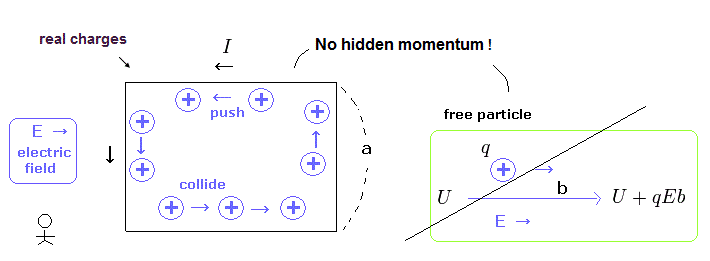In Fig.28, we treat each charge in the current as a free particle, when calculating the kinetic energy change.
But in the uniform electric current, each charge is not independent from each other, as shown in Fig.36.
So the momentum of Fig.33 is not true.

And if these positive charges in the current gain energy when they are flowing in the x direction, from the electric field E, these charges push the whole wire in the x direction.
( Pushing power is stronger in the x direction than in -x direction due to the energy difference. )

(Fig.37)But in fact, the negative charges inside the wire are pulled in the opposite direction by the electric field, and cancel the positive charge's energy.
Because negative and positve charges inside the wire pull each other.

## Other methods for getting hidden momentum.

(Fig.38) Start from torque = 0 in S frame.This paper introduces another method of getting hidden momentum of Fig.33.
In S frame, external magnetic field B is zero.

So the total torque on the whole wire having magnetic momentu μ is zero ( μ × B = 0).
Under Lorentz transformation, this relation of μ×B changes like Fig.38 right.

They define the part of μE/c2 as hidden momentum.
Of course, this hidden momentum is NOT a real thing, at all.

(Fig.39) Lorentz transformation of electromagnetic fields.The Lorentz transformation of electromagnetic field is Fig.39.
The electric field E is parallel to the observer's velocity, so the magnetic field B is always zero due to cross pruduct.

So they use the precondition of torque = 0, from the beginning.

(Fig.40) Torque in S and S' frames.Using Fig.39, the torques in S (= Fig.40 left ) and S' (= Fig.40 right ) frames are given by Fig.40.
Of course, these torques are always zero, from the beginning.

The electric field E in the x direction does NOT change under Lorentz transformation ( E = E' ).
And the magnetic momentu μ is considered to be equal in both frames.

(Fig.41)Using Fig.41, Fig.40 becomes

(Fig.42) Torque in S' frame is zero ?The first term includes electric dipole moment (= p ), which causes ordinary torque, as I said above.
They artificially define the second term contains hidden momentum, which can cancel the first term.

But this derivation is based on the precondition of N = 0 in Fig.40.
Lorentz transformation of "zero" becomes "zero", it is quite natural.
So, this method does NOT give solutions to the above paradox at all.

## Fictitious center is indispensable for hidden momentum.

(Fig.43) Virtual center of rotation is indispensable.The velosity "v" included in the second term of Fig.42 originates from virtual radius r = Vt.
The time derivative of angular momentum gives torque.

They use the fictitious relation of Fig.43 lower to get torque.
As I said many times, this virtual center is NOT real.

## Electric hidden momentum. ← artificial concept.

In Fig.44, there are external electric filed E and the magnetic moment of the current.
So the momentum of the electromagnetic field ( E × H ) exits.

But these electromagnetic energy and momentum are pseudoenergy.
"Real" energy and momentum exist inside the electric wire and capacitor, because the Coulomb energy needs the "real" charges ( more than one ).

The magnetic moment can be expressed as
(Fig.44)where H means magnetic field intensity, and B means magnetic flux density.

So the hidden momentum in the electromagnetic field becomes
(Fig.45)So using Fig.45, they insist the increased angular momentum caused by the observer's movement is cancelled out by Fig.34.

Of course, this hidden momentum can NOT stop the rotation of the wire.
Because the external electric field E acts on the generated charges themselves.
This pseudomomentum has nothing to do with it.

If this constant electromagnetic flow ( E × H) in the -y direction is real, where is the source of this flow ? This is strange.
And as I said, the torque of the internal rotation of the wire is NOT related to the whole momentum.2014/5/25 updated. Feel free to link to this site.